# Ellipsometer

## Contents

### Overview

An elipsometer is used measure the dielectric properties (including refractive index and dielectric function) of thin films. It is useful for determining these properties

• film thickness
• refractive indices
• surface roughness
• interfacial mixing
• composition
• crystallinity
• anisotropy
• uniformity

### Significance

The ellipsometer directly measures Ψ the amplitude ratio and Δ the phase difference of the polarization of light as it reflects off a sample surface. The data is collected at several incident angles and across a broad spectrum of wavelengths. Given these parameters the data is then fit to a set of mathematical models that is appropriate for the materials or multiple layers of materials. Once the other parameters are adjusted and the curves are properly fit, the desired property can be inferred.$\rho$ (a complex quantity), which is the ratio of$r_p$ over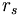$r_s$: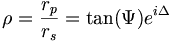$\rho = \frac{r_p}{r_s} = \tan ( \Psi ) e^{i \Delta}$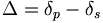$\Delta = \delta_p - \delta_s\,\!$ is the phase difference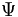$\Psi\,\!$ is the amplitude ratio

Other common variables associated with this are;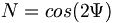$N= cos(2\Psi)\,\!$$C= sin(2\Psi) cos (\Delta)\,\!$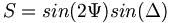$S= sin(2\Psi) sin (\Delta)\,\!$

Kinds of polarization simulation

This simulations shows linear, circular and elliptical polarization. Click the buttons to select a phase delay for the the waves and then press play.